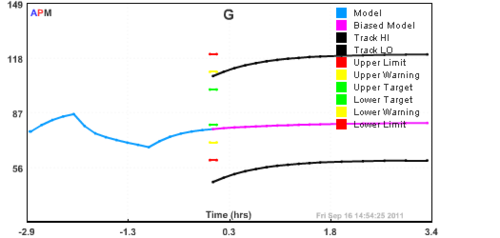## Blood Glucose Response of an Insulin Dependent Patient

This is a collection of mathematical models that predict the blood glucose levels of a type-I diabetic. These models have been used in research for blood glucose control. The objective is to predict the relationship between insulin injection and blood glucose levels. With a sufficiently accurate mathematical model of a patient, the correct insulin injection rate could be prescribed. By automating the sensing of blood glucose and the injection of insulin, this system would serve as an artificial pancreas. The following mathematical models are composed of differential and algebraic equations.

### Blood Glucose Simulation with PythonA. Roy and R.S. Parker. Dynamic Modeling of Free Fatty Acids, Glucose, and Insulin: An Extended Minimal Model, Diabetes Technology and Therapeutics 8(6), 617-626, 2006.

S. M. Lynch and B. W. Bequette, Estimation based Model Predictive Control of Blood Glucose in Type I Diabetes: A Simulation Study, Proc. 27th IEEE Northeast Bioengineering Conference, IEEE, 2001.

and

S. M. Lynch and B. W. Bequette, Model Predictive Control of Blood Glucose in type I Diabetics using Subcutaneous Glucose Measurements, Proc. ACC, Anchorage, AK, 2002.


! APMonitor Modeling Language
! https://www.apmonitor.com
!
! Model source:
! A. Roy and R.S. Parker. Dynamic Modeling of Free Fatty
!   Acids, Glucose, and Insulin: An Extended Minimal Model,
!   Diabetes Technology and Therapeutics 8(6), 617-626, 2006.
!
Model human
Parameters
p1 = 0.068       ! 1/min
p2 = 0.037       ! 1/min
p3 = 0.000012    ! 1/min
p4 = 1.3         ! mL/(min * micro-U)
p5 = 0.000568    ! 1/mL
p6 = 0.00006     ! 1/(min * micro-mol)
p7 = 0.03        ! 1/min
p8 = 4.5         ! mL/(min * micro-U)

k1 = 0.02        ! 1/min
k2 = 0.03        ! 1/min
pF2 = 0.17       ! 1/min
pF3 = 0.00001    ! 1/min
n = 0.142        ! 1/min
VolG = 117       ! dL
VolF = 11.7      ! L

! basal parameters for Type-I diabetic
Ib = 0           ! Insulin (micro-U/mL)
Xb = 0           ! Remote insulin (micro-U/mL)
Gb = 98          ! Blood Glucose (mg/dL)
Yb = 0           ! Insulin for Lipogenesis (micro-U/mL)
Fb = 380         ! Plasma Free Fatty Acid (micro-mol/L)
Zb = 380         ! Remote Free Fatty Acid (micro-mol/L)

! insulin infusion rate
u1 = 3           ! micro-U/min

! glucose uptake rate
u2 = 300         ! mg/min

! external lipid infusion
u3 = 0           ! mg/min
End Parameters

Intermediates
p9 = 0.00021 * exp(-0.0055*G)  ! dL/(min*mg)
End Intermediates

Variables
I = Ib
X = Xb
G = Gb
Y = Yb
F = Fb
Z = Zb
End Variables

Equations
! Insulin dynamics
$I = -n*I + p5*u1 ! Remote insulin compartment dynamics$X = -p2*X + p3*I

! Glucose dynamics
$G = -p1*G - p4*X*G + p6*G*Z + p1*Gb - p6*Gb*Zb + u2/VolG ! Insulin dynamics for lipogenesis$Y = -pF2*Y + pF3*I

! Plasma Free Fatty Acid (FFA) dynamics
$F = -p7*(F-Fb) - p8*Y*F + p9 * (F*G-Fb*Gb) + u3/VolF ! Remote FFA dynamics$Z = -k2*(Z-Zb) + k1*(F-Fb)
End Equations

End Model